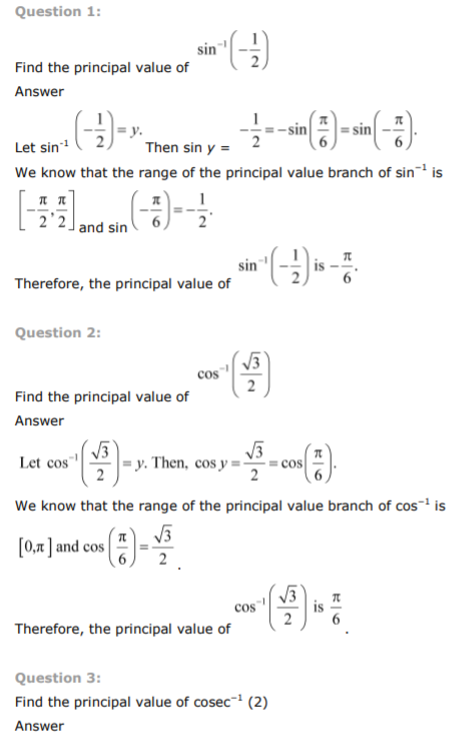# NCERT Solutions Class 12 Mathematics Chapter 2 Inverse Trigonometric Functions

## Chapter 2 Inverse Trigonometric Functions Class 12 Mathematics NCERT Solutions

Class 12 Mathematics students should refer to the following NCERT questions with answers for Inverse Trigonometric Functions in standard 12. These NCERT Solutions with answers for Grade 12 Mathematics will come in exams and help you to score good marks

### Inverse Trigonometric Functions Chapter 2 NCERT Solutions Class 12 Mathematics

MATHSNCERT Solutions Class 12 Mathematics Chapter 1 Relations and Functions
 NCERT Solutions Class 12 Mathematics Chapter 2 Inverse Trigonometric Functions
 NCERT Solutions Class 12 Mathematics Chapter 3 Matrices
 NCERT Solutions Class 12 Mathematics Chapter 4 Determinants
 NCERT Solutions Class 12 Mathematics Chapter 5 Continuity and Differentiability
 NCERT Solutions Class 12 Mathematics Chapter 6 Application of Derivatives
 NCERT Solutions Class 12 Mathematics Chapter 7 Integrals
 NCERT Solutions Class 12 Mathematics Chapter 8 Application of Integrals
 NCERT Solutions Class 12 Mathematics Chapter 9 Differential Equations
 NCERT Solutions Class 12 Mathematics Chapter 10 Vector Algebra
 NCERT Solutions Class 12 Mathematics Chapter 11 Three Dimensional Geometry
 NCERT Solutions Class 12 Mathematics Chapter 12 Linear Programming
 NCERT Solutions Class 12 Mathematics Chapter 13 Probability

Tags